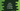# Java program to check if a user input number is strong or not## Java program to check if a user input number is strong or not:

In this post, we will learn how to check if a user input number is strong number or not in Java. A number is called a strong number if the sum of all factorials of its digits is equal to the number itself.

For example, let’s check for 145, It’s sum of factorials of each digit is : 1! + 4! + 5! = 1 + 24 + 120 = 145, i.e. the number itself. So, 145 is a strong number.

Our program will ask the user to enter a number. It will check if it is strong or not and print a message.

### Java Program:

Below is the complete Java program that checks for a number if it is a strong number or not:

``````import java.util.Scanner;

public class Main {

static int getFactorial(int digit) {
int fact = 1;
for (int j = digit; j > 1; j--) {
fact *= j;
}
return fact;
}

static boolean isItStrong(int userInputNo) {
int no = userInputNo;
int sum = 0;
while (no > 0) {
int digit = no % 10;
sum += getFactorial(digit);

no = no / 10;
}
return sum == userInputNo;
}

public static void main(String[] args) {
Scanner sc = new Scanner(System.in);
System.out.println("Enter a number to check : ");

int no = sc.nextInt();

if(isItStrong(no)){
System.out.println("Entered number is a strong number");
}else{
System.out.println("Entered number is not a strong number");
}
}
}``````

### Explanation:

• getFactorial method takes one number and return the factorial for it.
• isItStrong method checks if a number is strong or not. This is the method that we will use to check for a user input number. This method uses a while loop and gets the rightmost digit using % 10. For that digit, it gets the factorial value and adds it to a variable sum. Finally it checks if the value of sum is equal to the given number or not. If yes, it returns true, else returns false.
• Inside main, we are asking the user to enter a number to check for strong. The entered number is stored in the variable no.
• We are checking if it is a strong number or not using isItStrong. Based on the return value, we are printing one message if it is strong or not.

### Output:

It will print output as like below:

``````Enter a number to check :
146
Entered number is not a strong number

Enter a number to check :
145
Entered number is a strong number``````